## Example Questions

← Previous 1 3

### Example Question #1 : Geometry

Based on the table below, when x = 5, y will equal

 x y -1 3 0 1 1 -1 2 -3

11

–9

–11

–10

–9

Explanation:

Use 2 points from the chart to find the equation of the line.

Example: (–1, 3) and (1, –1)

Using the formula for the slope, we find the slope to be –2.  Putting that into our equation for a line we get y = –2x + b.  Plug in one of the points for x and y into this equation in order to find b.  b = 1.

The equation then will be: y = –2x + 1.

Plug in 5 for x in order to find y.

y = –2(5) + 1

y = –9

### Example Question #1 : Geometry

What is the slope of a line that runs through points: (-2, 5) and (1, 7)?

2

5/7

3/2

2/3

2/3

Explanation:

The slope of a line is defined as a change in the y coordinates over a change in the x coordinates (rise over run).

To calculate the slope of a line, use the following formula: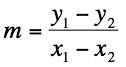### Example Question #3 : Geometry

A line passes through the points (–3, 5) and (2, 3). What is the slope of this line?

2/3

-3/5

–2/3

2/5

–2/5

–2/5

Explanation:

The slope of the line that passes these two points are simply ∆y/∆x = (3-5)/(2+3) = -2/5

### Example Question #4 : Geometry

Which of the following lines intersects the y-axis at a thirty degree angle?

y = x

y = x√2 - 2

yx((√3)/3) + 1

y = x√3 + 2

y = x - √2

y = x√3 + 2

Explanation: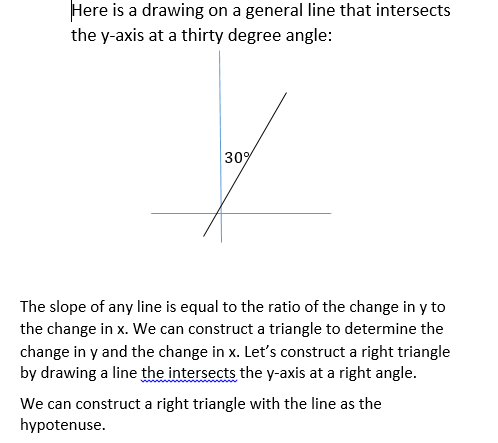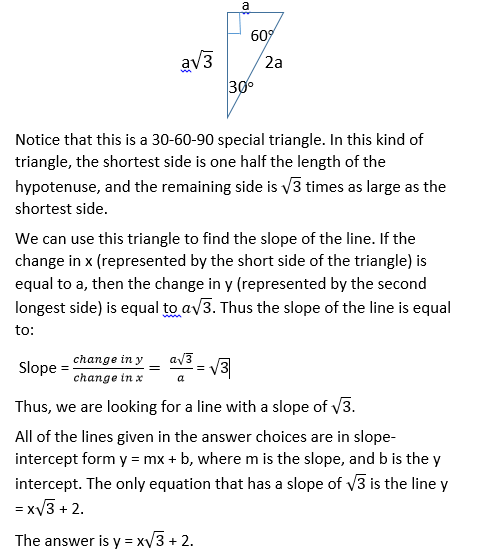### Example Question #5 : Geometry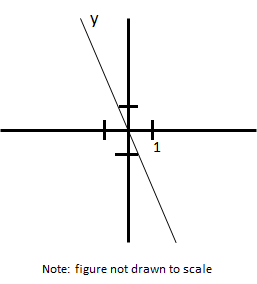What is a possible slope of line y?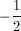2

–2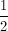–2

Explanation:

The slope is negative as it starts in quadrant 2 and ends in quadrant 4. Slope is equivlent to the change in y divided by the change in x. The change in y is greater than the change in x, which implies that the slope must be less than –1, leaving –2 as the only possible solution.

### Example Question #6 : Geometry

What is the slope between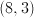and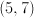?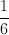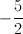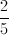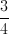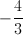Explanation:

Let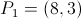and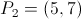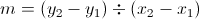so the slope becomes.

### Example Question #8 : How To Find The Slope Of A Line

What is the slope of line 3 = 8y - 4x?

2

0.5

-0.5

-2

0.5

Explanation:

Solve equation for y. y=mx+b, where m is the slope

### Example Question #9 : How To Find The Slope Of A Line

Find the slope of the line  6X – 2Y = 14

-6

-3

12

3

3

Explanation:

Put the equation in slope-intercept form:

y = mx + b

-2y = -6x +14

y = 3x – 7

The slope of the line is represented by M; therefore the slope of the line is 3.

### Example Question #1 : How To Find The Slope Of A Line

If 2x – 4y = 10, what is the slope of the line?

0.5

–5/2

–0.5

2

–2

0.5

Explanation:

First put the equation into slope-intercept form, solving for y: 2x – 4y = 10 → –4y = –2x + 10 → y = 1/2*x – 5/2. So the slope is 1/2.

### Example Question #1 : How To Find The Slope Of A Line

What is the slope of the line with equation 4x – 16y = 24?

1/2

–1/8

1/8

1/4

–1/4

1/4

Explanation:

The equation of a line is:

y = mx + b, where m is the slope

4x – 16y = 24

–16y = –4x + 24

y = (–4x)/(–16) + 24/(–16)

y = (1/4)x – 1.5

Slope = 1/4

← Previous 1 3